A radioactive material loses 10% of its mass each year. What proportion will be left there after n=6 years?

x =  0.5314

### Step-by-step explanation: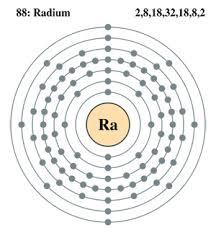Did you find an error or inaccuracy? Feel free to write us. Thank you!Tips to related online calculators1000$is invested at 10% compound interest. What factor is the capital multiplied by each year? How much will be there after n=12 years? • The town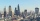The town population is 56000. It is decreasing by 2% every year. What will be the population of the town after 13 years? • The half lifeThe half-life of a radioactive isotope is the time it takes for a quantity of the isotope to be reduced to half its initial mass. Starting with 145 grams of a radioactive isotope, how much will be left after 3 half-lives? • Profit growthThe profit of a company increased by 25% during the year 1992, increased by 40% during the year 1993, decreased by 20% in 1994 and increased by 10% during the year 1995. Find the average growth in the profit level over the four years periods? • Volume of woodEvery year, at the same time, an increase in the volume of wood in the forest is measured. The increase is regularly p% compared to the previous year. If in 10 years the volume of wood has increased by 10%, what is the number p? • Account operationsMy savings of php 90,000 in a bank earns 6% interest in a year. If i will deposit additional php 10,000 at the end of 6 months, how much money will be left if i withdraw php 25,000 after a year? • Car valueThe car loses value 15% every year. Determine a time (in years) when its price will halve. • DepositIf you deposit 719 euros at the beginning of each year, how much money we have at 1.3% (compound) interest after 9 years? • Annual interest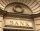A loan of 10 000 euro is to be repaid in annual payments over 10 years. Assuming a fixed 10% annual interest rate compounded annually, calculate: (a) the amount of each annual repayment (b) the total interest paid. • PopulationWhat is the population of the city with 3% annual growth, if in 10 years the city will have 60,000 residents? • Machine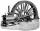Price of the new machine is € 62000. Every year is depreciated 15% of residual value. What will be the value of the machine after 3 years? • The crime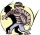The crime rate of a certain city is increasing by exactly 7% each year. If there were 600 crimes in the year 1990 and the crime rate remains constant each year, determine the approximate number of crimes in the year 2025. • The city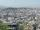At the end of 2010 the city had 248000 residents. The population increased by 2.5% each year. What is the population at the end of 2013? • SavingsThe depositor regularly wants to invest the same amount of money in the financial institution at the beginning of the year and wants to save 10,000 euros at the end of the tenth year. What amount should he deposit if the annual interest rate for the annua • If you 3If you deposit$4500 at 5% annual interest compound quarterly, how much money will be in the account after 10 years?The town has 65,000 inhabitants. 40 years ago, there were 157,000. How many people will live in a city in 10 years if the population's average rate is as in previous years?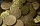The annual depreciation of the machine is 10%. After 8 years, the machine is worth 697 euros. What was the price of this machine at the time of purchase?